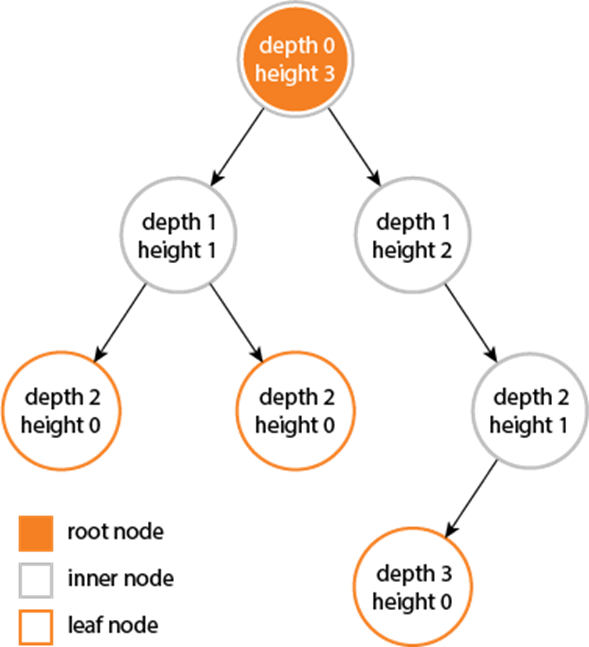# Height and Depth of Binary Tree

In this tutorial, we will learn how to find height and depth of binary tree with program implementation in C++. It is one of the most commonly used non-linear data structures. We will learn about:

• What is the height of a binary tree?
• Algorithm and implementation for finding height of Binary tree
• What is the depth of a binary tree?
• Algorithm and implementation for finding depth of Binary tree

Many times, people are confused between Depth and Height of Binary tree. It is because the depth of binary tree is always equal to the height of binary tree but they are not the same and using the terms interchangeably is not correct. So, it is important for us to understand the difference between the Height and Depth of Binary tree.## Height of Binary Tree

“Dream as high as the sky and as Deep as the ocean.”

As the quote on top says sky is what we should see while calculating height. The height of binary tree is the measure of length of the tree in the vertical direction. It is measured in upward direction that is from child to parent. The leaf nodes have height of 0 as there is no nodes below them. The height of the root node of the binary tree is the height of the whole tree. The height of a particular node is the number of edges on the longest path from that node to a leaf node.

Finding the Height of Binary Tree

To find the height of the binary tree we will recursively calculate the height of the left and right subtree of a node. To find the heights of left and right subtrees we use in-order traversal. After finding the height of both left and right subtree we will store the height of the subtree which has maximum value and add 1 to it to include the current level of tree.

### C++ Program to Find Height of Binary Tree

Output:

The Height of the binary tree is 3

## Depth of Binary Tree

Think of ocean and the quote above while calculating depth. The depth is a measure of how far a node is from the root of the tree. The depth of the ocean is calculated with respect to the sea level similarly the depth of any node in binary tree is measured with respect to the root node of the tree. The depth of a particular node in binary tree is the number of edges from the root node to that node. The depth of binary tree is the depth of the deepest node (leaf node).

To find the depth of the binary tree we will recursively calculate the depth of the left and right child of a node. After finding the depth of both left and right child we will store the depth of the child which has maximum value and add 1 to it to include the current level of tree.

### C++ Program to Find Depth of Binary Tree

Output:

The Depth of the binary tree is 3

Comment down below if you have queries related to height and depth of binary tree.

### 5 thoughts on “Height and Depth of Binary Tree”

1.Hi, in the diagram above, did you mean to say ‘height=2’ for the first left node of the root? It currently says ‘height=1’.

1.Nevermind. I think I understand it now.

2.Sunil Kublalsingh

Hi,

I’m pretty sure your image is wrong because the code used to find the height should result in having a leaf node with height of 1, NOT 0.

3.Sunil Kublalsingh

Your findHeight and findDepth functions are exactly the same. The only difference is the variable names and comments.

4.Sunil Kublalsingh

Why did you subtract 1 from the findDepth result. Shouldn’t the function just give you the answer? Shouldn’t it be the “depth of root” and not the “depth of root -1”